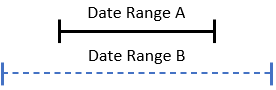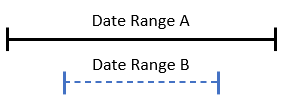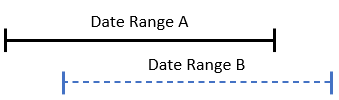In this article, we will discuss how to determine whether two date ranges overlap in C#. We will explore how to achieve this result using the DateOnly and TimeOnly records and then, we’re going to beautify our implementation with a custom class representing date ranges.

Let’s start it.

## Method to Determine Whether Two Date Ranges Overlap

First, let’s create a new console application using the `dotnet new console` through CLI or we can use the Visual Studio Wizard.

Now that we have the application, let’s check how to determine whether the two date ranges overlap.

To accomplish this, let’s create a method to compare dates:

```public static bool Overlap(DateOnly startDate1, DateOnly endDate1, DateOnly startDate2, DateOnly endDate2)
{
return startDate1 < endDate2 && startDate2 < endDate1;
}```

First, this method takes four `DateOnly` parameters, with the first two representing the start and end of the first date range, and the last two representing the start and end of the second time frame.

Then, we compare if the `startDate1` is less than `endDate2` and `startDate2` is less than `endDate1`. If both conditions are true, then we have an overlap date range and we should return `true`, otherwise, we return `false`.

It is important to mention that, if the second date range starts at the same time as the end of the first date range, we are not considering an overlap. However, if we need to consider it an overlap, we simply change the less than symbol (`<`) to less or equal than (`<=`) in both comparisons.

To learn more about the format of DateTimes, check out our great article on DateTime Format in C#.

## How Do Two Date Ranges Overlap?

Before we run the `Overlap()` method, let’s examine the first scenario in which two date ranges may intersect.

The first scenario is when the duration of the first date is entirely encompassed within the time frame of the second date:Let’s run the `Overlap()` method covering this scenario:

```var firstScenario = OverlapChecker.Overlap(new(2023, 01, 06),
new(2023, 01, 12),
new(2023, 01, 04),
new(2023, 01, 14));

Console.WriteLine(firstScenario); //true```

The second scenario covers the opposite situation. In this case, the start and end dates of the second date range fall within the first date range:Now, let’s change the parameters and call the `Overlap()` method to cover the second scenario:

```var secondScenario = OverlapChecker.Overlap(new(2023, 01, 04),
new(2023, 01, 14),
new(2023, 01, 06),
new(2023, 01, 12));

Console.WriteLine(secondScenario); //true```

Then we have the third scenario that occurs when the start date of the first range is before the start date of the second range, but the end of the first range falls between the start and end dates of the second range:Let’s run the `Overlap()` method against two date ranges to cover the third scenario expecting `true` as a result:

```var thirdScenario = OverlapChecker.Overlap(new(2023, 01, 04),
new(2023, 01, 12),
new(2023, 01, 07),
new(2023, 01, 15));

Console.WriteLine(thirdScenario); //true```

Finally, in the last scenario, the start date of the second range precedes the start date of the first range, but its end date falls within the time frame of the first range:Let’s run the `Overlap()` method passing the fourth scenario date ranges as parameters:

```var fourthScenario = OverlapChecker.Overlap(new(2023, 01, 07),
new(2023, 01, 15),
new(2023, 01, 04),
new(2023, 01, 12));

Console.WriteLine(fourthScenario); // true```

To finish, let’s create a fifth scenario, but this time, the date range is not going to overlap:

```var fifthScenario = OverlapChecker.Overlap(new(2023, 01, 07),
new(2023, 01, 15),
new(2023, 01, 16),
new(2023, 01, 22));

Console.WriteLine(fifthScenario); //false```

As we can see, this time we are passing two date ranges as parameters, and the second date range starts after the end of the first date range.

## Determine Whether Two Time Ranges Overlap

It is worth mentioning that we are using the `DateOnly` record that is available since .NET 6. But we can apply the same principle in case we want to use a `TimeOnly` or `DateTime` class.

Let’s create a new method to check if two time ranges overlap:

```public static bool OverlapTime(TimeOnly startTime1, TimeOnly endTime1, TimeOnly startTime2, TimeOnly endTime2)
{
return startTime1 <= endTime2 && startTime2 <= endTime1;
}```

The only difference is that we replaced the `DateOnly` record with `TimeOnly` record.

Now, let’s run this method with an overlap time range:

```var overlapTimeRange = OverlapChecker.OverlapTime(new TimeOnly(10, 00),
new TimeOnly(12, 00),
new TimeOnly(11, 00),
new TimeOnly(15, 00));

Console.WriteLine(overlapTimeRange); //true```

Now, let’s run it against a scenario in which the `OverlapTime()` method returns `false`:

```var dontOverlapTimeRange = OverlapChecker.OverlapTime(new(10, 00),
new TimeOnly(12, 00),
new TimeOnly(15, 00),
new TimeOnly(16, 00));

Console.WriteLine(dontOverlapTimeRange); //false```

## Improve the Solution With DateRange Class

The `Overlap()` method above solves our problem, however, we can make our solution more attractive with a `DateRange` class:

```public class DateRange
{
public DateOnly Start { get; private set; }
public DateOnly End { get; private set; }

public DateRange(DateOnly start, DateOnly end)
{
Start = start;
End = end;
}

public bool Overlap(DateRange range)
{
return Start < range.End && End > range.Start;
}
}```

The `DateRange` class represents a range of dates between a start and an end date.

It contains two `DateOnly` public properties, `Start` and `End`, to store the start and end dates.

This class constructor takes two `DateOnly` instances as a parameter to set the value of the `Start` and `End` properties.

Additionally, the `DateRange` class includes a public `Overlap()` method which takes another `DateRange` object as a parameter. This method is responsible for checking if the current DateRange object overlaps with the input `DateRange` instance.

To determine whether these two date ranges overlap, we compare if the start `DateOnly` of the current instance is less than the end of the input date range and if the end of the current `DateRange` is greater than the start of the input parameter `DateRange`.

In case of overlapping date ranges, the method returns true. Otherwise, it returns false.

Now that the `DateRange` class is ready, let’s run it in a scenario in which the date ranges overlap:

```var firstOverlapDateRange = new DateRange(new(2023, 01, 06), new(2023, 01, 12));
var secondOverlapDateRange = new DateRange(new(2023, 01, 04), new(2023, 01, 14));

Console.WriteLine(firstOverlapDateRange.Overlap(secondOverlapDateRange)); //true```

As expected, the `Overlap()` method returns `true`.

And let’s run it against a scenario in which the date ranges don’t overlap:

```var firstDontOverlapDateRange = new DateRange(new(2023, 01, 07), new(2023, 01, 15));
var secondDontOverlapDateRange = new DateRange(new(2023, 01, 16), new(2023, 01, 22));

Console.WriteLine(firstDontOverlapDateRange.Overlap(secondDontOverlapDateRange)); //false```

## Conclusion

In this article, we have learned how to determine whether two date ranges overlap in C#, as well as explore the TimeOnly variation.

First, we have seen the four ways a date range might overlap with another date range. Then, we checked how to implement the logic to check whether two date ranges overlap. Finally, we have created a DateRange class to make our solution more attractive.